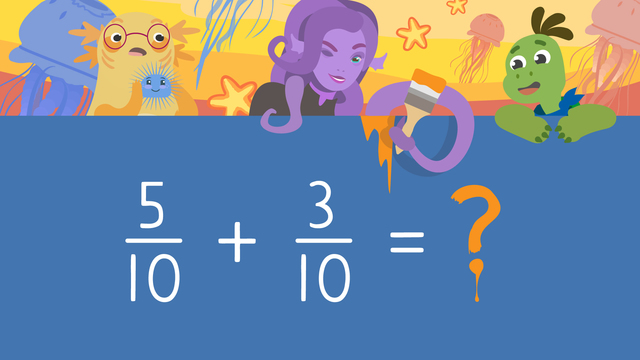# Adding Fractions with Like DenominatorsRate this video

Ø 5.0 / 1 ratings

The authorTeam Digital
CCSS.MATH.CONTENT.4.NF.B.3.A

### Contents

Axel and Tank follow the sounds of crying into a cave. They discover that Ophelia the Sea Witch is trying to decorate for her party and has made a big mess! In order to help Ophelia we need to learn about and practice adding Fractions with like denominators.

### How to Add Fractions with Like Denominators

Adding fractions with the same denominators is similar to addition with whole numbers, but instead we are adding simple fractions. When adding fractions with common denominators, there are some steps to adding fractions that need to be followed. To add fractions there are Three Simple Steps:

• Step 1: Check that the denominators are the same, and write it in the total
• Step 2: Add the numerators
• Step 3: Write the numerator above the denominator in the total

These steps are the rules for adding fractions with like denominators. Many have asked “when adding fractions do you add the denominator?” and the answer is no. The number of parts your whole is broken into does not change, so we never add the denominators when adding two fractions.

Let’s take a look at an example of adding fractions with same denominator and adding fractions and simplifying. Here, we’ll add five-tenths plus three-tenths following the steps and rules for adding fractions with like denominators.

• Step 1: Check that the denominators are the same, and write it in the total• Step 2: Add the numerators• Step 3: Write the numerator above the denominator in the totalLast, we can simplify eight-tenths into four-fifths.### How to Add Fractions with Like Denominators Summary

When adding fractions with the same denominators, we keep in mind the rules for adding fractions with like denominators. To add fractions there are Three Simple Steps:

• Step 1: Double check that the denominators are the same, and write it in the total
• Step 2: Add the numerators
• Step 3: Write the numerator above the denominator in the total

Want some more adding fractions practice? In this video you can find adding fractions examples and on this website there is adding fractions with like denominators worksheets along with other activities, and exercises.

### TranscriptAdding Fractions with Like Denominators

Can you hear that sound? It sounds like Ophelia, the sea witch, crying inside of her lair! Axel and Tank NEED to know what the fuss is about... ...and they find that Ophelia is trying to get ready for a party, but has made a big mess! She needs help counting the jellyfish, starfish, and sea urchin decorations she is painting for a party. Let's practice [title] "adding fractions with like denominators" to count the decorations she is painting. A fraction describes a part of a whole OR a part of a collection.

The top number, or the NUMERATOR, represents how many equal parts of a whole we have. The bottom number, or the DENOMINATOR, represents how many equal parts our whole is divided into. (...) A COMMON or LIKE denominator refers to fractions with the SAME denominators. To start, Ophelia paints THREE out of SEVEN, or three-sevenths, of her jellyfish orange. If she paints two more, how many jellyfish will be painted total? (...) We can find the TOTAL by solving the equation three-sevenths PLUS two-sevenths. When you add fractions with LIKE denominators... the denominator ALWAYS stays the same since the number of parts in our whole does NOT change. That is why our first step is to double check that the denominators are the SAME. Since they are LIKE denominators we can write the denominator of our total HERE. The second step is to add the numerators. Three plus two is five, and we write it HERE. (...) That means, five-sevenths of Ophelia's jellyfish will be painted orange. Next, she paints five-tenths of the starfish yellow. If she paints three more, how many starfish will be painted total? (...) This time we will add five-tenths PLUS three-tenths. What is the first step? (...) First, double check that the denominators are the same, (...) and write it HERE. What is the second step? (...) The second step is to add the numerators. What is five plus three? (...) The sum is eight. (...) Eight-tenths can be simplified to four-fifths... which means, eight tenths OR four-fifths of Ophelia's starfish will be painted yellow. Finally, she paints four-twelfths of the sea urchins red... and she will paint eight more. (...) What is four-twelfths PLUS eight-twelfths? (...) First, double check that the denominators are the same, (...) and write it HERE. Next, add four plus eight to get (...) twelve. Twelve-twelfths can be simplified to one whole... which means Ophelia will paint ALL of her sea urchins red! Remember, (...) when adding fractions with LIKE denominators... The first step is to "double check that the denominators are the same, and write it in the total". When you add fractions with LIKE denominators, the denominators ALWAYS stay the same and DO NOT get added. The second step is to "add the numerators". The third step is to write the numerator above the denominator in the total, simplifying the fraction when you need to. Let's see how Ophelia's party turned out! Looks like she's the light of the party! [Ophelia is lit up on the ceiling as a decoration for the party as well]